﻿ Design of Stiffening Rings – Oil & Gas Industry Technology Updates

## Design of Stiffening Rings

The equations for the maximum strength of a cylindrical shell under external pressure are established under the assumption that the shell is simply supported. For this to be true, stiffening rings are used as lines of support, assumed to carry all the load that the shell carries due to external pressure. The size of the stiffening ring is calculated by using the equation for buckling of a circular ring under uniform external pressure.

The required moment of inertia of the stiffening ring is: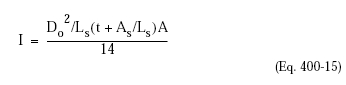where:
Ls = The sum of one-half the distances on both sides of the stiffening ring from the centerline of the ring to the (1) next stiffening ring, (2) to a circumferential line on the head line at one-third its depth, or (3) to a jacket connection.
As = Cross-sectional area of the stiffening ring

The ASME Code allows a portion of the shell to be considered as contributing to the moment of inertia. The width of the shell section is 1.1 Dot, and is considered as laying one-half on each side of the centroid of the ring.

In this case, the required moment of inertia of the combined area is: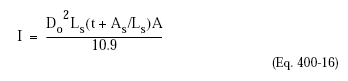The Code procedure for determining the size of the ring is as follows:

Step 1. Assume a ring size. Determine As
Step 2. Calculate Factor B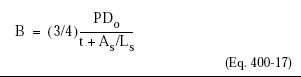Step 3. From the material chart in Appendix A, find the value of A.
Step 4. Calculate the value of Is. The moment of inertia of the ring or the combined section (ring plus shell section) has to be larger than the required moment of inertia.

Example. Using the same vessel as in the previous example, select a 5 x 3 x 3/8 angle ring with As = 2.86 in2 and I = 7.37 in4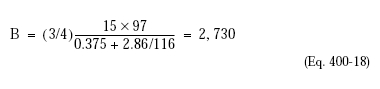From Figure UCS-282, A = 0.0002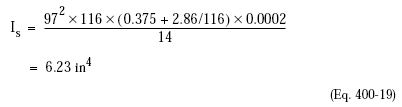Since the available moment of inertia is larger than the required moment of inertia, the vessel is adequately stiffened.